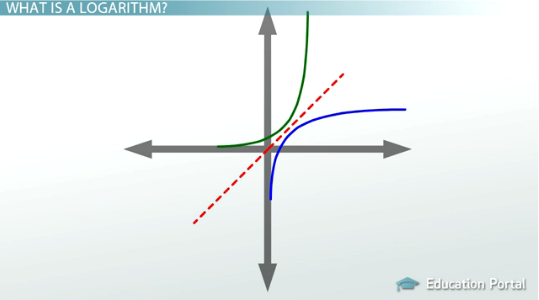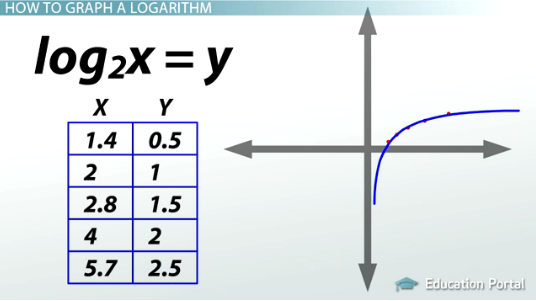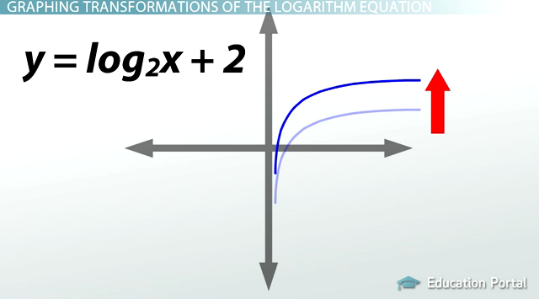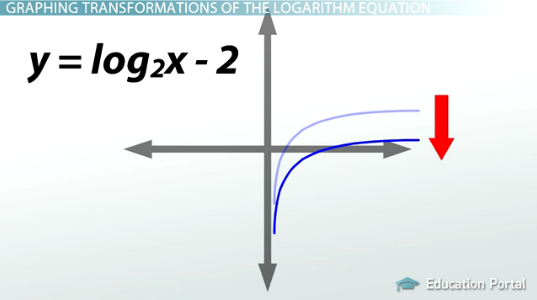# How to Graph Logarithms: Transformations and Effects on Domain/Range

An error occurred trying to load this video.

Try refreshing the page, or contact customer support.

Coming up next: How to Evaluate Logarithms

### You're on a roll. Keep up the good work!

Replay
Your next lesson will play in 10 seconds
• 0:06 What Is a Logarithm?
• 0:59 How to Graph a Logarithm
• 2:08 The Domain and Range…
• 2:44 Graphing…
• 4:58 The Effect of…
• 6:26 Lesson Summary
Save Save

Want to watch this again later?

Timeline
Autoplay
Autoplay
Speed Speed Audio mode

#### Recommended Lessons and Courses for You

Lesson Transcript
Instructor: Jennifer Beddoe

Jennifer has an MS in Chemistry and a BS in Biological Sciences.

The graph of a basic logarithm is relatively simple. This lesson will show you how to graph a logarithm and what the transformations will do to the graph as well as their effects on the domain and range of the graph.

## What Is a Logarithm?

The basic formula for a logarithm (log) is y = log2x is equivalent to 2y = x which means that the solution to a logarithm equation is the power you must raise a certain number to in order to obtain another number. The logarithm function is the inverse of an exponential, which is a term that has a variable in its exponent. For example, 2^x is an exponential.

It stands to reason, then, that the graph of a logarithm would be the inverse of the graph of an exponential. And you can see from this picture below that that is correct. The graph of the logarithm is just the graph of the exponential inverted across a straight line.## How to Graph a Logarithm

There are two methods you can use in order to graph a logarithm.

The first is to substitute in numbers for one variable to get values for the other, then plot them on a graph and connect the dots. When using this method, remember that the log is undefined at zero and less than zero, so x can only be greater than zero.

Let's graph the following as an example: log2x = y

The first step is to draw a chart, then fill in the values for x and y.

x y
1.4 0.5
2 1
2.8 1.5
4 2
5.7 2.5

You can pick any value for y that you desire, but smaller is usually better. This way your graph is not huge. You can get the general idea for the graph from 5 or so points.

Then, when you have your points, just plot them on the graph and connect the dots. Remember, with the graph of a general logarithm, it will never touch or cross the y-axis but will come as close as possible.The second method for graphing a logarithm is to use a graphing calculator. Read the instructions that came with your calculator in order to graph logarithms using this method.

## The Domain and Range of Logarithms

The domain of any equation is the possible x values for that equation. It is any number that x could possibly be when that equation is graphed on a coordinate plane. For the general logarithm equation, the domain is x > 0 because x cannot be zero or below.

The range, or possible y values for any equation, is any number that y could possibly be when the equation is graphed on the coordinate plane. For the general logarithm equation, the range is y = all real numbers.

## Graphing Transformations of the Logarithm Equation

So far, we have only talked about the general logarithm equation and its graph, but what if the equation is more complex, such as y = log2x + 2

Adding or subtracting to the equation causes the graph to shift, either up, down, left or right, depending on how the addition or subtraction is framed. Graphing logarithmic equations that have been shifted can be done very easily if you remember the set of rules that govern these shifts.

#### Rules for Graphing Transformations:

1. The first rule says that adding a number to the equation will cause the graph to shift up the number of spaces indicated by that number. The example shown previously has a +2 added to the equation, which means that the graph will shift up two spaces from the general graph.As you can see above, the graph has been shifted up two spaces.

2. The second rule states that subtracting a number from the equation will cause the graph to shift down that number of spaces. Take a look at this example: y = log2x - 2

Because of the -2 in the equation, the graph will be shifted down two spaces.To unlock this lesson you must be a Study.com Member.

### Register to view this lesson

Are you a student or a teacher?

#### See for yourself why 30 million people use Study.com

##### Become a Study.com member and start learning now.
Back
What teachers are saying about Study.com

### Earning College Credit

Did you know… We have over 200 college courses that prepare you to earn credit by exam that is accepted by over 1,500 colleges and universities. You can test out of the first two years of college and save thousands off your degree. Anyone can earn credit-by-exam regardless of age or education level.